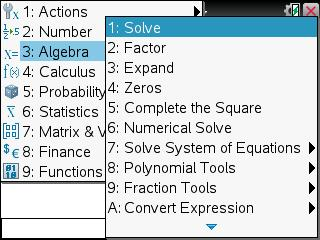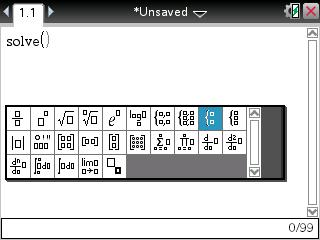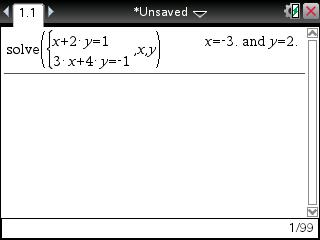# Knowledge Base

## Solution 24770: Solving a System of Linear Equations on the TI-Nspire™ Family.

### How do I simultaneously solve a system of linear equations on the TI-Nspire family?

Simultaneously solving a system of linear equations on the TI-Nspire family products can be done using the simult( command. The following example will solve x and y for the equations:

For Example:
x + 2y = 1
3x + 4y = -1

1) Press [home] [A] or add a Calculator app. This is the first option on the available icons at the bottom.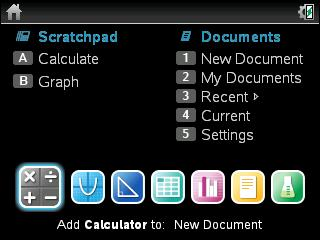2) Press [catalog] [S].
3) Scroll to simult( and press [enter].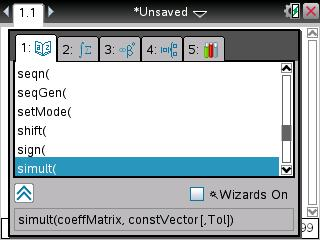4) Press [template]. This is the key located to the right of .
5) Scroll to the second row, sixth column and press [enter].
6) Press [del]  [tab] [del]  [enter].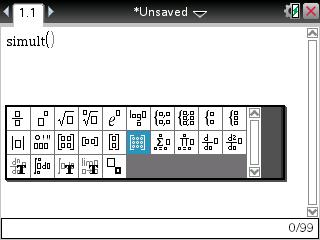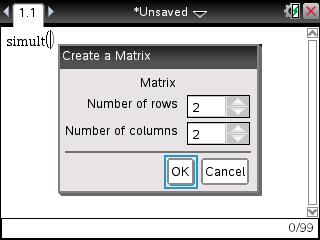7) Press  [tab]  [tab]  [tab]  [tab].
8) Press [,] [template].
9) Scroll to the second row, fifth column and press [enter].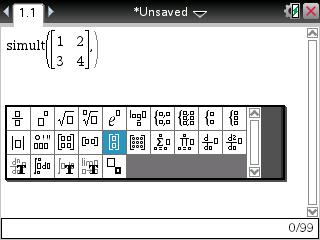10) Press  [tab] [(-)]  [enter].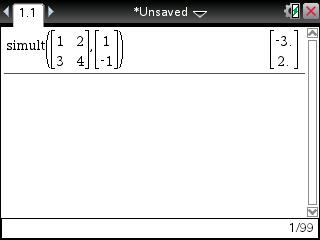TI-Nspire CAS family:

In addition to using the simult( command, the TI-Nspire CAS family can also use the solve( command and the simultaneous equation template to solve simultaneous equations: